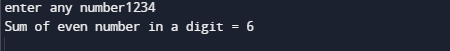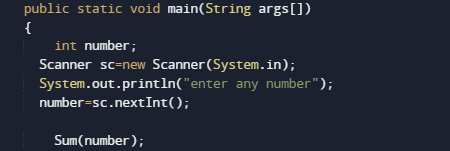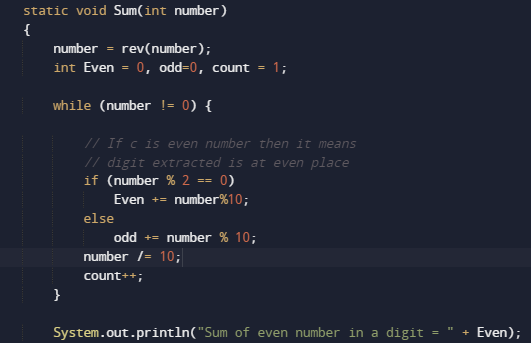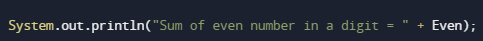# Java Program to Find sum of even digits in a number

In this tutorial you will learn about the Java Program to Find sum of even digits in a number and its application with practical example.

In this tutorial, we will learn to create a Java Program to Find sum of even digits in a number using Java programming.

## Prerequisites

Before starting with this tutorial we assume that you are best aware of the following Java programming topics:

• Java Operators.
• Basic Input and Output function in Java.
• Class and Object in Java.
• Basic Java programming. Basic Java programming.
• If-else statements in Java.
• For loop in Java.

## Even number

Even number are that number  that can be divided by two and gives remainder equals to zero.
Example: (Number/2==0) =>  (4/2==0) so 4 is a even Number.
Some even numbers are 2, 4, 6, 8, 10,……

## Odd Number

Odd numbers that are not divisible by 2. and does not give remainder = Zero, when divided by 2, some the odd numbers are end in 1, 3, 5, 7, 9, 11……

## Java Program to Find sum of even digits in a number

In this program we will learn to create a program that will find sum of even digits in a number. Firstly we declare required header file and variable and also initiates required values in variable. Next we take value from user at run time and then after we will find the sum of even digits in a number.

## OutputIn the above program, we have first declared and initialized a set variables required in the program.

• number= it will hold entered number.
• Even = it will hold sum or even digit.
• rev = for reversing a string.Here we first declared and initialized set of variables required in the program. Then we will take a value from user.After that we pass the given number to function named Sum().where we going to calculate sum of all even digit in a given number .Steps for adding even values.

1. Take a number from user,.
2. Declare a variable Even to store the sum of even value in a number.
3. Reverse a Digit to find last digit of the number.
4. Now check all the digit is even or not.
5. If it even then adds it to Even variable, else go to next step
6. Remove last digit of the number.
7. Repeat this until the number becomes 0.
8. And last print the sum of all even digit in a number.In this tutorial we have learn about the Java Program to Find sum of even digits in a number and its application with practical example. I hope you will like this tutorial.